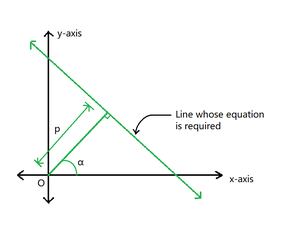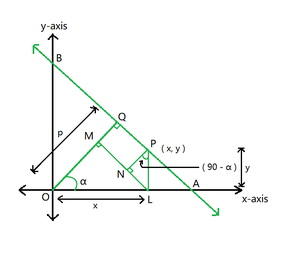Open In App

# Equation of a straight line with perpendicular distance D from origin and an angle A between the perpendicular from origin and x-axis

Given two integers D and A representing the perpendicular distance from the origin to a straight line and the angle made by the perpendicular with the positive x-axis respectively, the task is to find the equation of the straight line.

Examples:

Input: D = 10, A = 30 degrees
Output: 0.87x +0.50y = 10

Input: D = 12, A = 45 degrees
Output: 0.71x +0.71y = 12

Approach: The given problem can be solved based on the following observations:Figure 1

• Let the perpendicular distance be (p) and the angle between the perpendicular and the positive x-axis be (?) degrees.
• Consider a point P with coordinates (x, y) on the required line.
• Draw a perpendicular from P to meet the x-axis at L.
• From L, draw a perpendicular on OQ at M.
• Now, draw a perpendicular from P to meet ML at N.Figure 2

Now consider right triangle OLM— (1)

Now consider right triangle PNL— (2)

NowUsing equations (1) and (2)which is the equation of the required line

Below is the implementation of the above approach :

## C++

 `// C++ program for the approach``#include ``using` `namespace` `std;` `// Function to find equation of a line whose``// distance from origin and angle made by the``// perpendicular from origin with x-axis is given``void` `findLine(``int` `distance, ``float` `degree)``{``    ``// Convert angle from degree to radian``    ``float` `x = degree * 3.14159 / 180;` `    ``// Handle the special case``    ``if` `(degree > 90) {``        ``cout << ``"Not Possible"``;``        ``return``;``    ``}` `    ``// Calculate the sin and cos of angle``    ``float` `result_1 = ``sin``(x);``    ``float` `result_2 = ``cos``(x);` `    ``// Print the equation of the line``    ``cout << fixed << setprecision(2)``         ``<< result_2 << ``"x +"``         ``<< result_1 << ``"y = "` `<< distance;``}` `// Driver Code``int` `main()``{``    ``// Given Input``    ``int` `D = 10;``    ``float` `A = 30;` `    ``// Function Call``    ``findLine(D, A);` `    ``return` `0;``}`

## Java

 `// Java program for the approach` `class` `GFG{` `// Function to find equation of a line whose``// distance from origin and angle made by the``// perpendicular from origin with x-axis is given``static` `void` `findLine(``int` `distance, ``float` `degree)``{``    ``// Convert angle from degree to radian``    ``float` `x = (``float``) (degree * ``3.14159` `/ ``180``);` `    ``// Handle the special case``    ``if` `(degree > ``90``) {``        ``System.out.print(``"Not Possible"``);``        ``return``;``    ``}` `    ``// Calculate the sin and cos of angle``    ``float` `result_1 = (``float``) Math.sin(x);``    ``float` `result_2 = (``float``) Math.cos(x);` `    ``// Print the equation of the line``    ``System.out.print(String.format(``"%.2f"``,result_2)+ ``"x +"``         ``+ String.format(``"%.2f"``,result_1)+ ``"y = "` `+  distance);``}` `// Driver Code``public` `static` `void` `main(String[] args)``{``    ``// Given Input``    ``int` `D = ``10``;``    ``float` `A = ``30``;` `    ``// Function Call``    ``findLine(D, A);` `}``}` `// This code is contributed by shikhasingrajput`

## Python3

 `# Python3 program for the approach``import` `math` `# Function to find equation of a line whose``# distance from origin and angle made by the``# perpendicular from origin with x-axis is given``def` `findLine(distance, degree):` `    ``# Convert angle from degree to radian``    ``x ``=` `degree ``*` `3.14159` `/` `180`` ` `    ``# Handle the special case``    ``if` `(degree > ``90``):``        ``print``(``"Not Possible"``)``        ``return`` ` `    ``# Calculate the sin and cos of angle``    ``result_1 ``=` `math.sin(x)``    ``result_2 ``=` `math.cos(x)`` ` `    ``# Print the equation of the line``    ``print``(``'%.2f'` `%` `result_2,``          ``"x +"``, ``'%.2f'` `%` `result_1,``          ``"y = "``, distance, sep ``=` `"")` `# Driver code` `# Given Input``D ``=` `10``A ``=` `30` `# Function Call``findLine(D, A)` `# This code is contributed by mukesh07`

## C#

 `// C# program for the approach``using` `System;``class` `GFG``{``    ` `    ``// Function to find equation of a line whose``    ``// distance from origin and angle made by the``    ``// perpendicular from origin with x-axis is given``    ``static` `void` `findLine(``int` `distance, ``float` `degree)``    ``{``        ``// Convert angle from degree to radian``        ``float` `x = (``float``)(degree * 3.14159 / 180);``     ` `        ``// Handle the special case``        ``if` `(degree > 90) {``            ``Console.WriteLine(``"Not Possible"``);``            ``return``;``        ``}``     ` `        ``// Calculate the sin and cos of angle``        ``float` `result_1 = (``float``)(Math.Sin(x));``        ``float` `result_2 = (``float``)(Math.Cos(x));``     ` `        ``// Print the equation of the line``        ``Console.WriteLine(result_2.ToString(``"0.00"``) + ``"x +"``             ``+ result_1.ToString(``"0.00"``) + ``"y = "` `+ distance);``    ``}` `  ``static` `void` `Main ()``  ``{``    ``// Given Input``    ``int` `D = 10;``    ``float` `A = 30;`` ` `    ``// Function Call``    ``findLine(D, A);``  ``}``}` `// This code is contributed by suresh07.`

## Javascript

 ``

Output:

`0.87x +0.50y = 10`

Time Complexity: O(1)
Auxiliary Space: O(1)